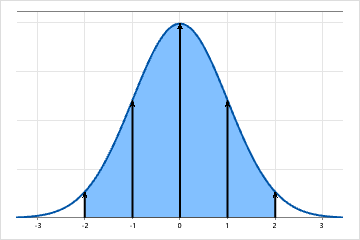# Normal distribution

The normal distribution is a continuous distribution that is specified by the mean (μ) and the standard deviation (σ). The mean is the peak or center of the bell-shaped curve. The standard deviation determines the spread of the distribution.

For example, in the following graph of a normal distribution, approximately, 68% of observations are within +/- 1 standard deviation of the mean; 95% are within +/- 2 standards deviations of the mean (as shown by the shaded area); and 99.7% are within +/- 3 standard deviations of the mean.The normal distribution is the most common statistical distribution because approximate normality occurs naturally in many physical, biological, and social measurement situations. Many statistical analyses assume that the data come from approximately normally distributed populations.